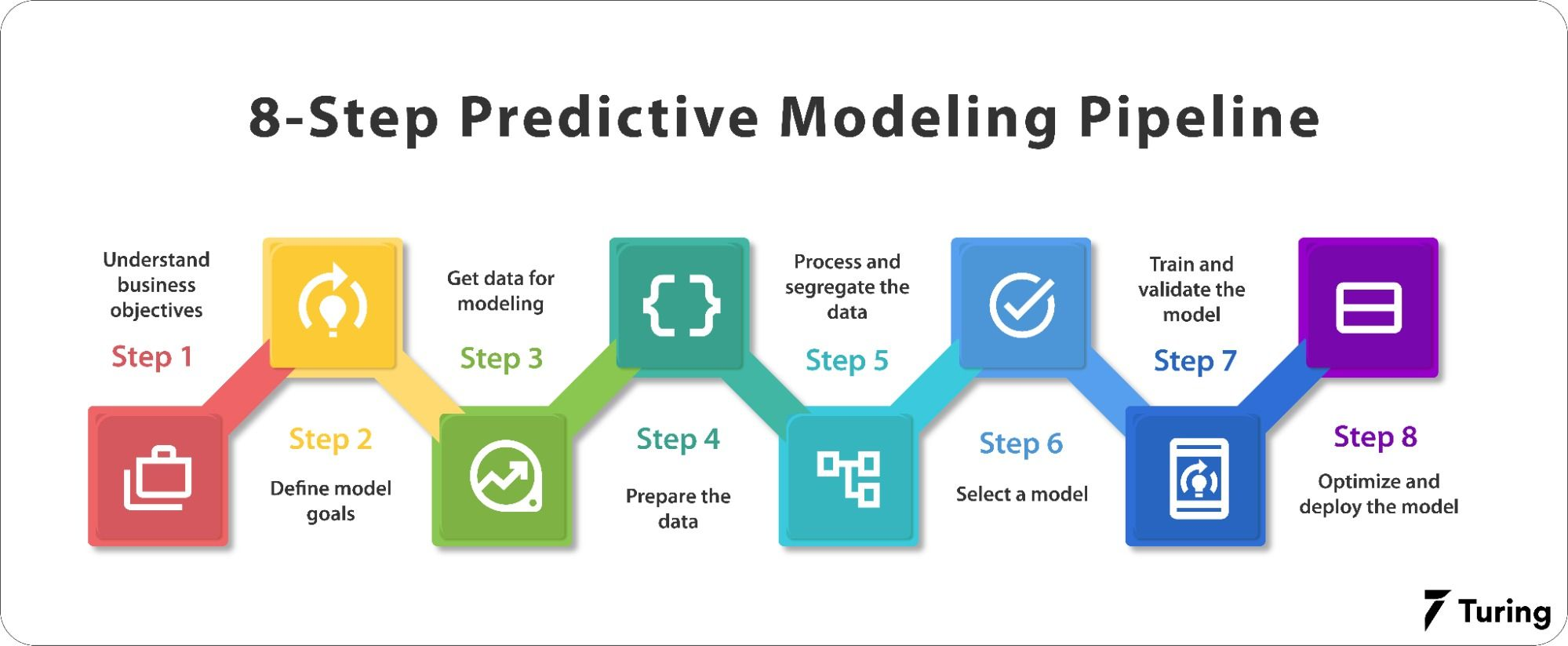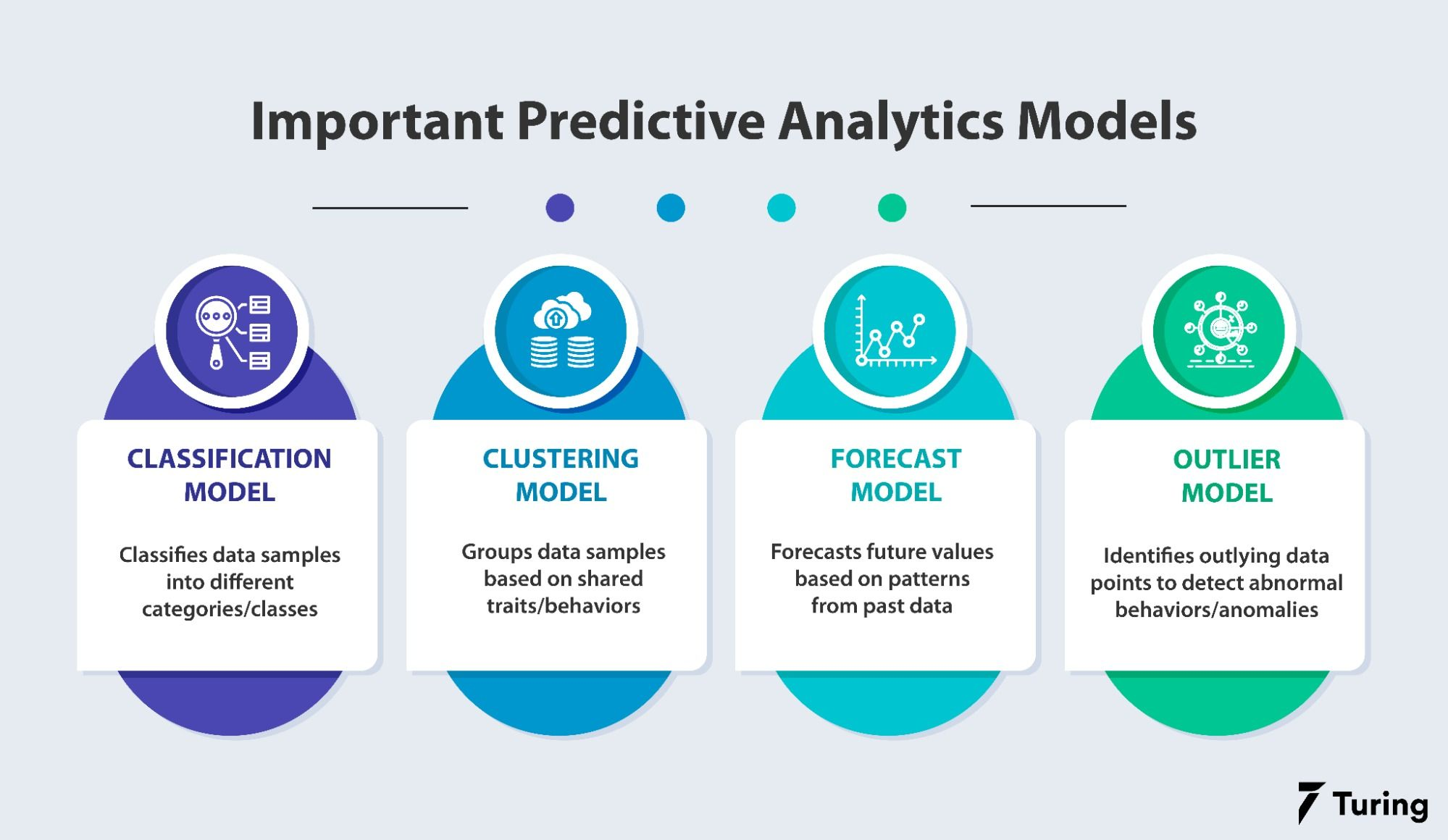# All You Have to Know About Predictive ModelingPredictive modeling is a mathematical and statistical method to predict future behavior or trends by analyzing the patterns of input data. It is a part of predictive analytics and uses current and historical data to predict or forecast future events. Some examples include predicting a stock's behavior over time or the price of a commodity like gold.

Although predictive modeling focuses on forecasting future trends, the process also aids in predicting a certain outcome based on the patterns learned from input data. This can predict the probability of, say, whether an email is spam or if a transaction is fraudulent. In these cases, the primary event has already happened but the model is used to determine its current state.

In this blog, we will dive deep into predictive modeling, explore various modeling techniques, and understand how to choose the right one for different situations. Let’s get started.

## Why is predictive modeling needed?

In predictive modeling, input data is collected on which a statistical model is trained, predictions are obtained, and the model is validated. The model helps provide valuable future insights and patterns about the case in question. This, in turn, gives companies a competitive advantage and lets them deliver the best services to their clients.

Following are some of the applications of predictive modeling:

• Supply chain management: Predictive modeling is used to predict the supply, demand, and costs in a company.

• Auto insurance: Predictive modeling can determine the risk of accidents to policyholders. It also helps determine insurance premiums depending upon the profile of policyholders.

• Fraud detection systems: Predictive modeling can identify high-risk transactions as fraud. It also identifies high-profile customers and aids in customer retention by enabling easier targeted customer service.

## The predictive modeling pipelineEvery predictive modeling approach follows a series of steps to enable an end-to-end fool-proof system. It can be summarized into an 8-step pipeline as described below:

The first step is to understand the objectives and necessities of the business in question. This includes details about the customers, market, and the business model of the client. Using these details, we define a particular problem.

### Defining model goals

In this step, we specify and define a problem statement in terms of predictive analytics which is solved using predictive modeling. We also decide on the distinct metrics that will be used to test the effectiveness of the model.

### Collecting data

Now that the goal and problem statement have been defined, we move on to collecting relevant data and creating the required dataset.

### Preparing the data

As the collected data is raw and unprocessed, we need to prepare it and organize it. This will enable us to build a better and more accurate predictive model.

### Processing and segregating the data

The collected data then needs to be processed statistically, i.e., the dependent and independent variables have to be separated. Required data processing operations like filling missing values, handling numerical and categorical values, etc., must also be performed before feeding the data to the model.

### Selecting the model

Now that we have the problem statement and the dataset ready, we can proceed with selecting a model. This depends on the type of modeling we are performing. For instance, whether it is regression, classification, forecasting, etc.

### Training and validation

This is the most important step of the predictive modeling process. Here, the selected model is trained with the processed dataset and validated on a separate validation dataset. The validation dataset is created using various cross-validation techniques like k-fold, stratified k-fold, and so on.

### Optimization and deployment

The trained model is optimized by testing it on different testing and validation datasets. The metrics of the model are maximized. It is then deployed into production where it provides results on real-world data.

## Types of predictive modelsThere are various modeling algorithms and techniques that can be used for different use cases. Some of the most important models are:

### Classification model

The classification model classifies the data sample into different categories or classes specified. Spam detection and fraud transaction detection are good examples of this category.

### Clustering model

The clustering model is a type of unsupervised predictive modeling approach. It groups data samples based on shared traits or behaviors. This helps companies to detect the behavior/class of new data samples when plotted with existing clusters.

A real-world example is predicting the credit risk for a loan applicant based on patterns of past data. Another is retail marketing, where marketers can use common features to analyze the spending habits and product interests among a group of customers for easier target advertising.

### Forecast model

The forecast model uses historical data of numerical values, i.e., stock prices, commodity prices, trends in real estate value, etc., and forecasts the future values based on patterns from past data. Some of the applications can be forecasting raw materials for manufacturing based on past monthly orders and supply chain statistics.

### Outlier model

The outlier model works by identifying outlying data points. This helps in identifying abnormal behaviors or anomalies. One of the best applications of this model is identifying a fraudulent/abnormal transaction based on the fact that it is not related to past spending habits and patterns.

We have discussed some of the fundamental types of predictive models used in real-world scenarios. Now, let’s explore commonly used algorithms and techniques to train the above models.

Algorithms used in predictive modeling are usually based on machine learning (ML) or deep learning (DL). Both are subsets of artificial intelligence (AI) with different applications. ML is applied to structured data like tabular data and numerical datasets. DL, which uses neural networks, is applied to unstructured data like images, audio, video, and text.

The following are some commonly used algorithms in predictive modeling:

### Random forest

A random forest model uses multiple decision trees to process vast amounts of data. It performs classification and regression analysis.

Gradient boosted algorithms like XGBoost and CatBoost are some of the best algorithms for working on structured data. These models work similarly to random forests, i.e., they use an ensemble of decision trees that work with each other to reduce the overall prediction error.

### K-means

K-means is a clustering model that groups data points based on similar features or properties. It can be used as a recommendation engine or anomaly detector.

### Generalized linear model (GLM) for two values

GLM is a generalization of ordinary linear regression. It reduces the number of variables to find the ‘best-fit’ line.

### Artificial neural network (ANN)

ANN is one of the most powerful algorithms used in predictive analytics. Note that a neural network requires large volumes of data to effectively find and compute patterns.

In this article, we explored what predictive modeling is, its necessity, and uses. We also learned about the predictive modeling pipeline and the different models and algorithms used. With this, we can conclude that in this information age where huge amounts of data are constantly generated every day - up to 2.5 quintillion bytes! - proper understanding of data is essential and predictive modeling can greatly help.

## Author

•### Turing

Author is a seasoned writer with a reputation for crafting highly engaging, well-researched, and useful content that is widely read by many of today's skilled programmers and developers.

Here are some factors that should be considered when choosing predictive modeling techniques:

• What does your target variable look like?
• Is there any issue with the computational performance?
• Do you plan to update your model with new data?
• Is your data linearly separable?
• Can your dataset fit into memory?

The prediction accuracy is measured by dividing the correct number of predictions with the total number of predictions. It provides an accurate prediction percentage.

We can leverage residuals to judge the quality of a model.### Press

What's up with Turing? Get the latest news about us here.### Blog